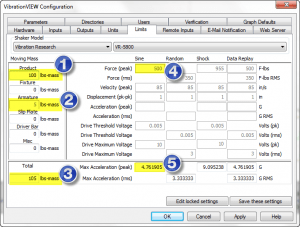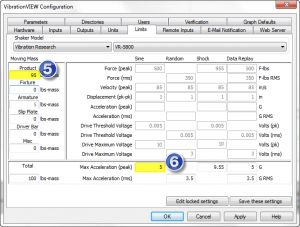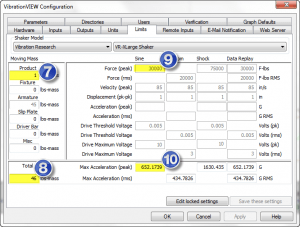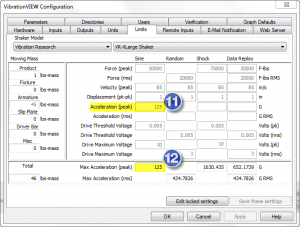# May the F=MA be with you.

#### Shakers

Force = Mass x Acceleration. This equation is the basis for sizing shakers and determining if your shaker can perform a specific shock or vibration test. The premise is this: the more mass you put on your shaker, the less acceleration the shaker can produce. And vice-versa: the less mass you put on your shaker, the more acceleration it can produce. You may have noticed that all shakers have a force rating, and it’s exactly these ratings that determine what combination of mass and acceleration can be achieved on a given shaker.

But wait a second! Have you ever found that you cannot reach the maximum acceleration based on the shaker’s force rating and the calculated F=MA? As an example:

• I have a 500 force-lb shaker
• I want to perform a 5g sine test
• My max product mass is 100lb (using M=F/A – 500 force-lb/5g)
• Run the test and my shaker can only reach 4.75g’s

It may seem that Force doesn’t always equal Mass x Acceleration. Why is this? The most common reason for this is due to the weight of the armature inside the shaker. When you add the weight of the armature and any other moving mass (such a driver bar when connecting a slip table to the armature), the maximum weight of your test item is reduced. The equation is correct, just remember to add up all of the moving masses.
VibrationVIEW software is a great tool for making these calculations automatically. We include many popular shaker models with their ratings pre-loaded for your convenience. Just enter the total moving mass and VibrationVIEW calculates the maximum acceleration possible for a given test type. Here is a screenshot of the software calculating the same set of conditions as above:We enter the product mass (field 1), armature mass (field 2) and the software adds up a total moving mass (field 3). With a shaker force of 500 force-lbs (field 4) the maximum acceleration is calculated (field 5). Here we can see that the maximum acceleration is less than 5g’s because the total moving mass is 105lbs. We now have to make a decision to either reduce our product mass or find a larger force shaker. If we reduce the product mass to 95lbs we can now reach our desired acceleration of 5g’s (fields 5 & 6 below).More on Shaker Limits. Shakers are designed to test products for shock and vibration and are sized based on some minimum expected level of moving mass. This is especially true when we speak about large force shakers. If we are testing a low mass product on a large force shaker and calculate for the maximum acceleration level we can come up with some very high maximum acceleration values. Here is an example:With a small product mass of 1 lb (field 7) our total moving mass is 46 lbs (field 8). Using a 30,000 force-lb shaker (field 9) the maximum calculated acceleration for sine testing is just over 650g’s. I would not be exaggerating if I said this is an insane number to test at! The calculation is correct, but the shaker has some limits on what acceleration levels it can perform at without damaging itself. This is typically dependent on the physical characteristics of the shaker/armature and the voltage/current capabilities of the amplifier. In this example the “real” maximum acceleration level of the shaker (designated by the shaker manufacturer) should not exceed 125g’s. VibrationVIEW software includes a field that allows the user to enter this maximum peak acceleration value, a sort of “never to exceed” value (see fields 11 & 12 below).Although the focus here has been on calculating for force, mass and acceleration, your shaker also has other limits for velocity, displacement (aka “stroke”) and drive voltage. VibrationVIEW software includes limit fields for these parameters as well. Always double-check your shaker manual for the correct ratings and when in doubt, contact the shaker manufacturer for final clarification. Once you have all of the correct ratings set up in VibrationVIEW it will warn you when you setup a test profile that is beyond your shaker’s capabilities.

Happy testing and may the F=MA be with you.

Date

February 6, 2018

Author

Jared

Category

Shakers

Share Via